﻿ PBO钻孔应变同震响应分析文章快速检索 高级检索
 大地测量与地球动力学2019, Vol. 39Issue (7): 765-770  DOI: 10.14075/j.jgg.2019.07.020### 引用本文CHEN Yifei, LÜ Pinji. Analysis of Coseismic Response of PBO Borehole Strainmeters[J]. Journal of Geodesy and Geodynamics, 2019, 39(7): 765-770.### Foundation support

Scientific Research Fund of Institute of Seismology and Institute of Crustal Dynamics, CEA, No. IS201526214.

### Corresponding author

LÜ Pinji, associate professor, majors in crustal deformation and earthquake, E-mail:pinjilv@163.com.

### 第一作者简介

CHEN Yifei, postgraduate, majors in crustal deformation data analysis, E-mail:yefychen@163.com.

### 文章历史

PBO钻孔应变同震响应分析

1. 中国地震局地震研究所地震大地测量重点实验室，武汉市洪山侧路40号，430071;
2. 中国地震局地壳应力研究所武汉创新基地，武汉市洪山侧路40号，430071

1 观测数据

2017-09-08墨西哥发生8.1级地震，USGS公布的发震时刻为UTC 04:49:19，震中为(15.022°N，93.899°W)，震源深度47.4 km。以PBO观测站记录的地震波数据为研究对象，选取钻孔应变仪与地震仪同址观测的14个站点(表 1)记录的地震波数据，对地震发生后1 h的连续观测数据进行分析。表 1 站点信息 Tab. 1 Site information
1.1 数据预处理

PBO钻孔应变仪(BSM)采集的原始数据是仪器四元件记录的电容值时间序列，需要对原始电容值g进行变换，转换为便于研究的线性拉伸应变e

 $e=R \frac{g / C}{1-g / C}$ (1)

1.2 降采样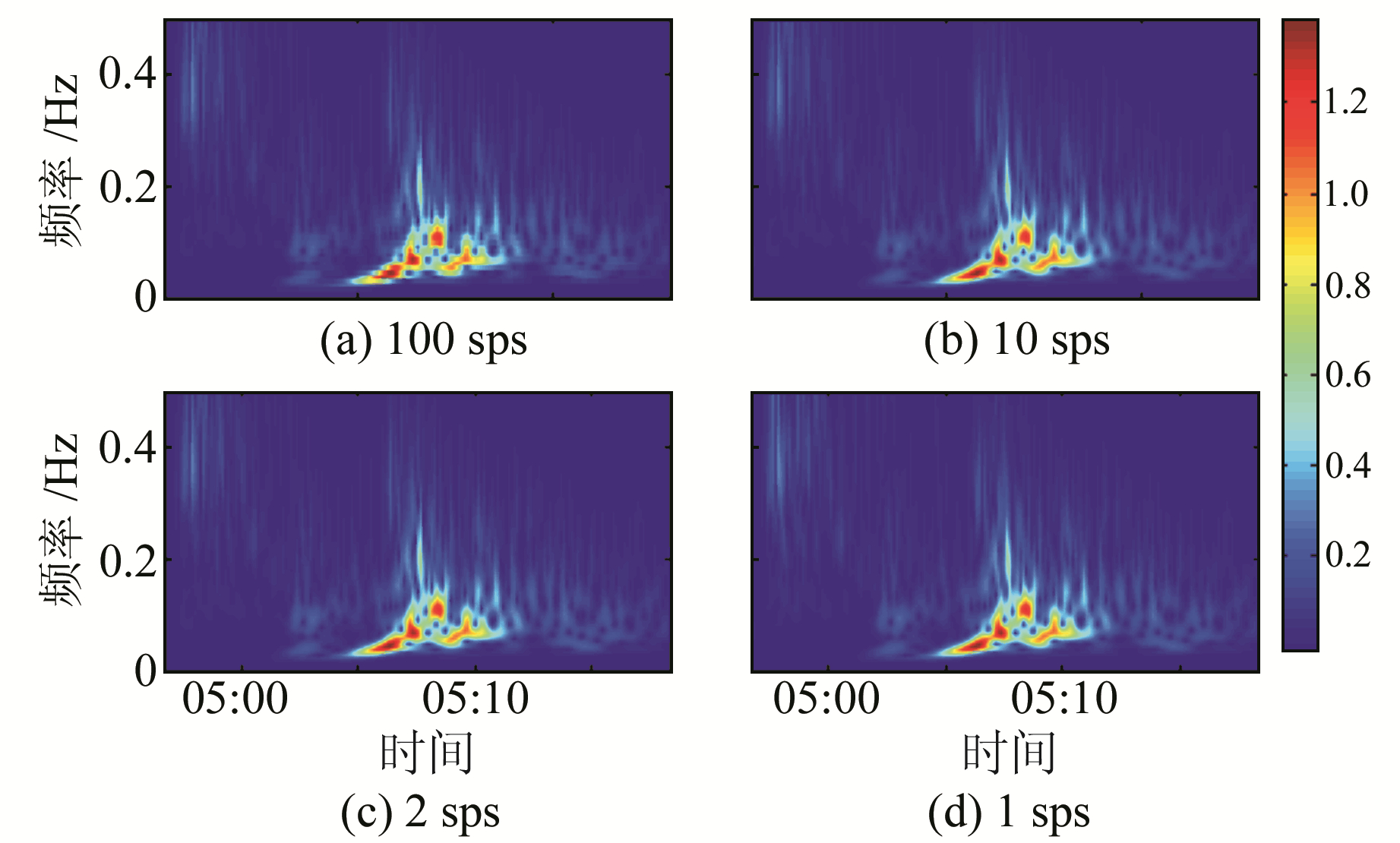图 1 地震计记录地震波降采样过程全波段S变换谱 Fig. 1 Seismometer recording drop sampling process S-transformation
 $\left\{ {\begin{array}{*{20}{l}} {S[\tau , f] = \sum\limits_{t = 0}^{N - 1} s [t]\exp \left[ { - \frac{{{{(\tau - t)}^2}{f^2}}}{2}} \right]{{\rm{e}}^{\frac{{ - 2{\rm{ \mathsf{ π} }}ift}}{N}}}}\\ {s[t] = \frac{1}{N}\sum\limits_{f = - \frac{N}{2}}^{\frac{N}{2} - 1} {\sum\limits_{f = 0}^{N - 1} S } [\tau , f]{{\rm{e}}^{\frac{{ - 2\pi ift}}{N}}}} \end{array}} \right.$ (2)

1.3 PBO钻孔应变仪的自检

PBO钻孔应变仪与国内的YRY-4应变仪都由4个元件按次序排列组成。YRY-4应变仪的元件在水平面按间隔45°分布，各元件观测记录为si，因此YRY-4自检符合以下关系：

 $S_{i}=k s_{i}$ (3)
 $S_{1}+S_{3}=S_{2}+S_{4}$ (4)

PBO钻孔应变仪k个元件在水平面排列顺序为60°、60°、30°。将PBO钻孔应变仪看作由元件组合成的两个子模块：CH0、CH1和CH2元件间隔60°，跨越角120°；CH1和CH3间隔90°。假设元件各分量相对权重是正确的，且仪器与围岩耦合良好，两个子模块提供了两种不同的元件组合模块，且两种子模块组合都占有分量元件CH1，则此两种不同的元件组合子模块彼此相等于面应变[10-11]

 $\frac{\left(g_{0} e_{0}+\mathrm{g}_{1} e_{1}+g_{2} e_{2}\right)}{3}=C \varepsilon_{a}$ (5)
 $\frac{\left(g_{1} e_{1}+g_{3} e_{3}\right)}{2}=C \varepsilon_{a}$ (6)表 2 自检相关系数 Tab. 2 Correlation coefficient
1.4 射线路径参数

USGS公布的墨西哥8.1级大地震的震源深度为47.4 km。表 3记录的是在该震源深度下震中距从26°~ 43°的理论P波射线参数(模型为IASP91，表 3中“射线参数1”)，在该震源深度下，其相应的震中距对应的射线路径参数(表 3中“射线参数2”)为12.3~13.8 km/s。表 3 震中距射线路径参数 Tab. 3 Epicentral distance and ray path parameters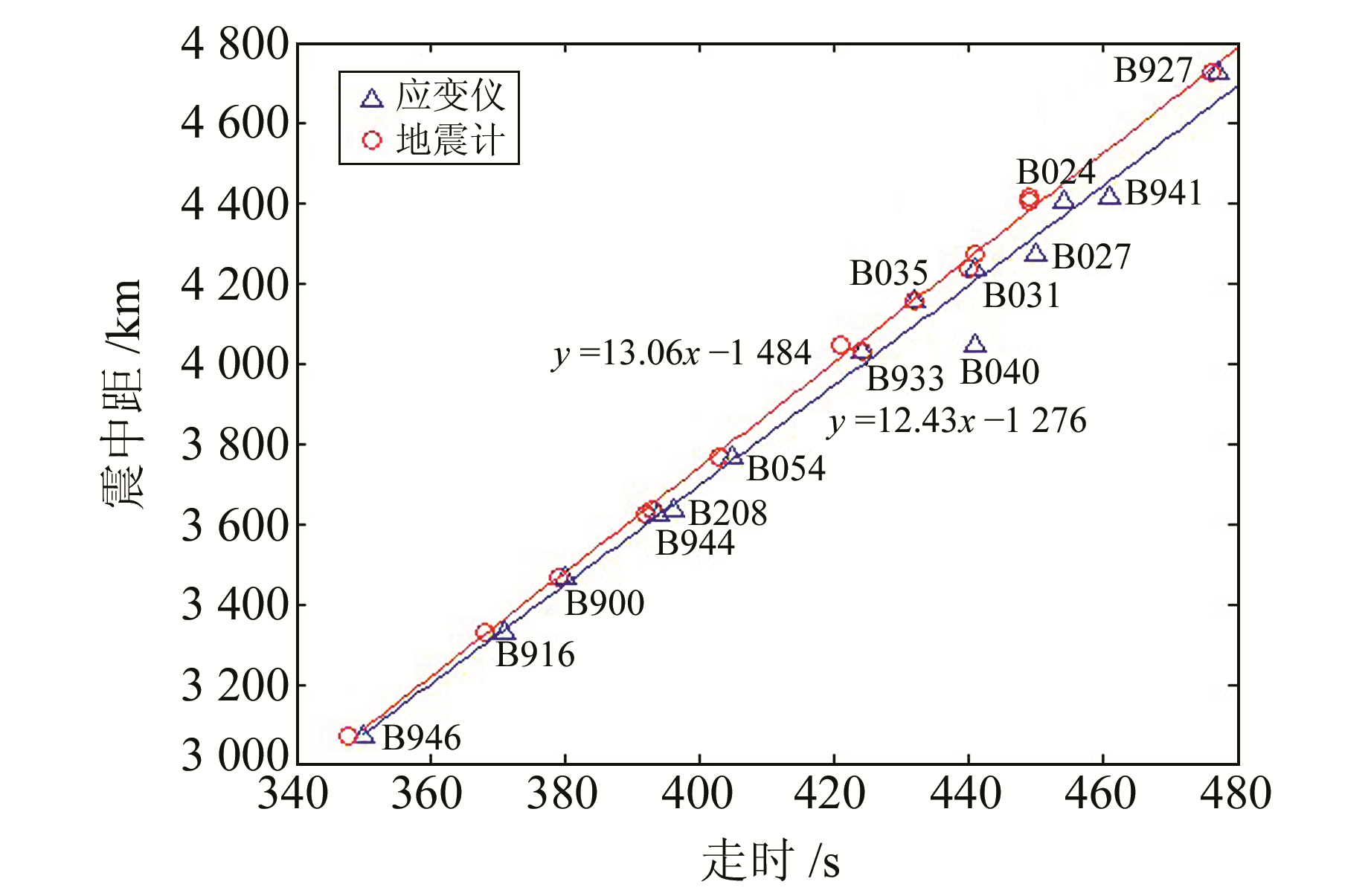图 2 仪器P波走时与震中距拟合曲线 Fig. 2 Fitting curves of P wave travel time and epicentral distance
2 频谱分析比较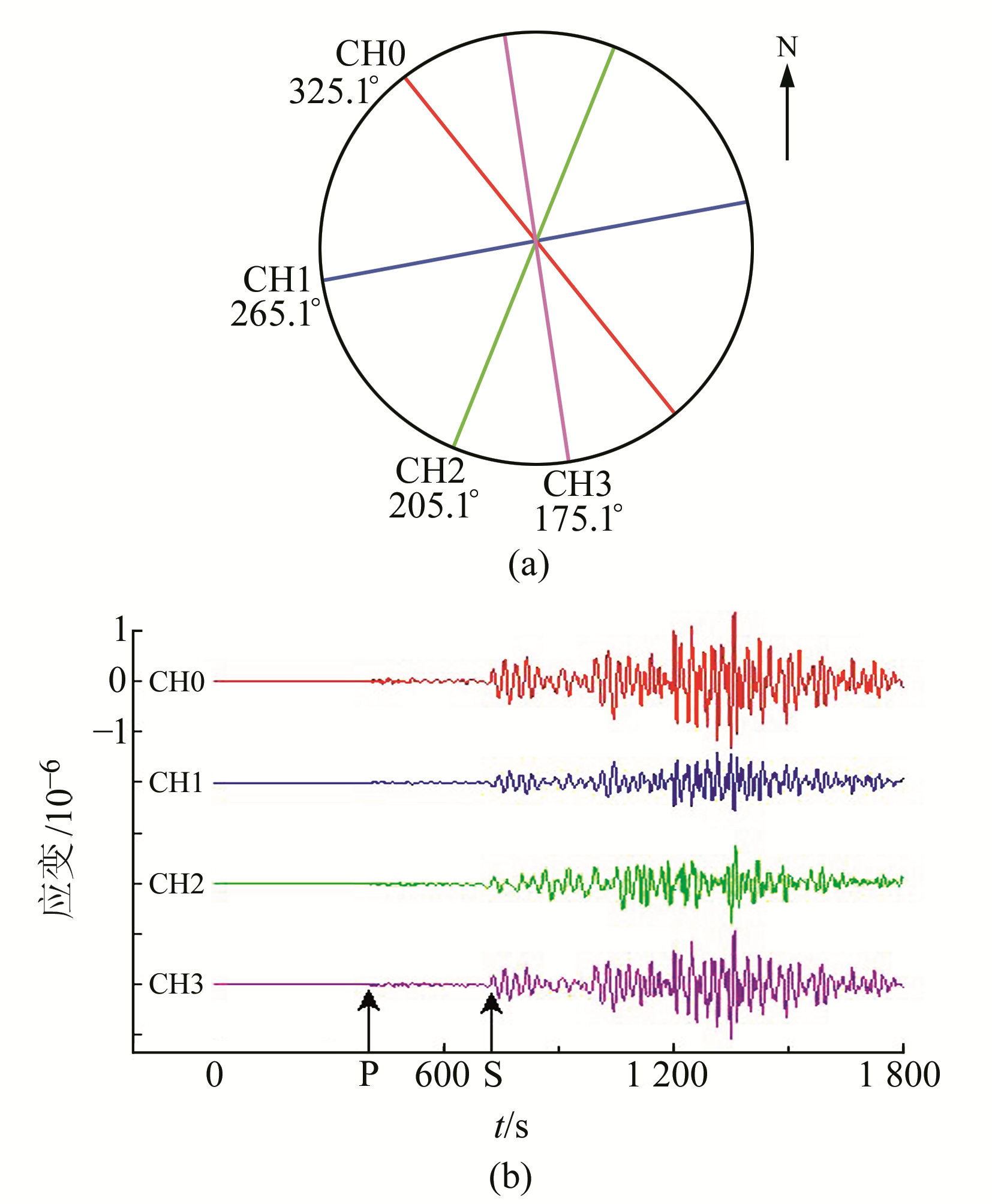图 3 PBO钻孔应变网B944站点应变时间序列(各元件颜色与观测记录对应) Fig. 3 Time series of strain from B944 in PBO borehole strain network(the color of each component corresponds to the observation record)
2.1 波形整体特征分析图 4 站点应变仪地震波记录S变换 Fig. 4 The S-transform of strainmeter earthquake records for B946, B944 and B941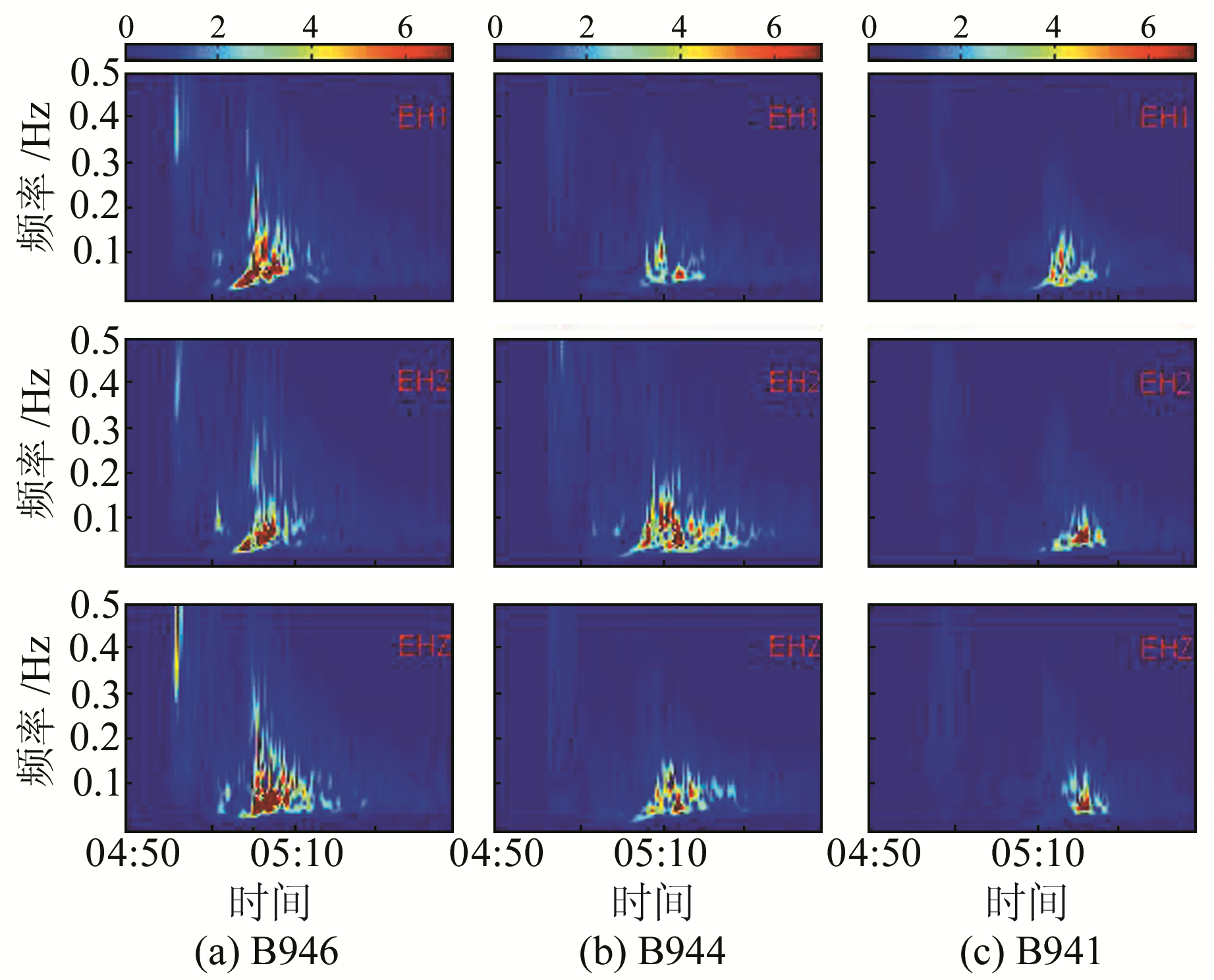图 5 站点地震仪地震波记录S变换，B946、B944、B941震中距依次增大(EH1和EH2为水平分量，EHZ为垂向分量) Fig. 5 Seismograph record's S transform: B946, B944, B941, which epicenter distance increases in turn (EH1 and EH2 are horizontal components, EHZ is vertical component)
2.2 波段比较分析

S变换谱的P波段分析表明，钻孔应变仪记录的地震波优势频段为0.2 Hz，分量元件方位越接近震中连线，其记录的面波能量明显越强于其他分量。两种仪器记录的波形发育过程一致性较好。S波段的应变记录主要频段为0.1 Hz，与P波段相比其频率组分更偏向低频；地震仪的S波段频谱也偏向中低频，且连续性较好。

PBO钻孔应变仪记录的面波随着时间呈现由低频向高频转化的过程。这是由于低频信号能传播到较深的地层，传播介质速率较快从而先行到达；而高频面波的传播深度相对较浅，介质速率小而晚到达。随着时间的推移，应变仪面波主频率由0.02 Hz延伸到0.1 Hz，地震仪面波主频率由0.02 Hz延伸到0.12 Hz。地震仪在面波段的频谱与PBO应变仪响应较为一致地记录了面波的发育过程。

3 同震应变主方向

 $S_{\theta_{i}}=A\left(\varepsilon_{1}+\varepsilon_{2}\right)+B\left(\varepsilon_{1}-\varepsilon_{2}\right) \cos 2\left(\theta_{i}-\varphi\right)$ (7)

 \left\{\begin{aligned} S_{3}=& A\left(\varepsilon_{1}+\varepsilon_{2}\right)+B\left(\varepsilon_{1}-\varepsilon_{2}\right) \cos 2\left(\theta_{3}-\varphi\right) \\ S_{2}=& A\left(\varepsilon_{1}+\varepsilon_{2}\right)+\frac{1}{2} B\left(\varepsilon_{1}-\varepsilon_{2}\right) \cos 2\left(\theta_{3}-\varphi\right)-\\ & \frac{\sqrt{3}}{2} B\left(\varepsilon_{1}-\varepsilon_{2}\right) \sin 2\left(\theta_{3}-\varphi\right) \\ S_{1}=& A\left(\varepsilon_{1}+\varepsilon_{2}\right)-B\left(\varepsilon_{1}-\varepsilon_{2}\right) \cos 2\left(\theta_{3}-\varphi\right) \\ S_{0}=& A\left(\varepsilon_{1}+\varepsilon_{2}\right)+\frac{1}{2} B\left(\varepsilon_{1}-\varepsilon_{2}\right) \cos 2\left(\theta_{3}-\varphi\right)+\\ & \frac{\sqrt{3}}{2} B\left(\varepsilon_{1}-\varepsilon_{2}\right) \sin 2\left(\theta_{3}-\varphi\right) \end{aligned}\right. (8)

 $\left\{\begin{array}{l}{S_{02}=S_{0}-S_{2}} \\ {S_{13}=S_{1}-S_{3}}\end{array}\right.$ (9)

 $\varphi=\frac{1}{2} \arctan \left(\frac{2}{\sqrt{3}} \frac{S_{02}}{S_{13}}\right)+\theta_{3}$ (10)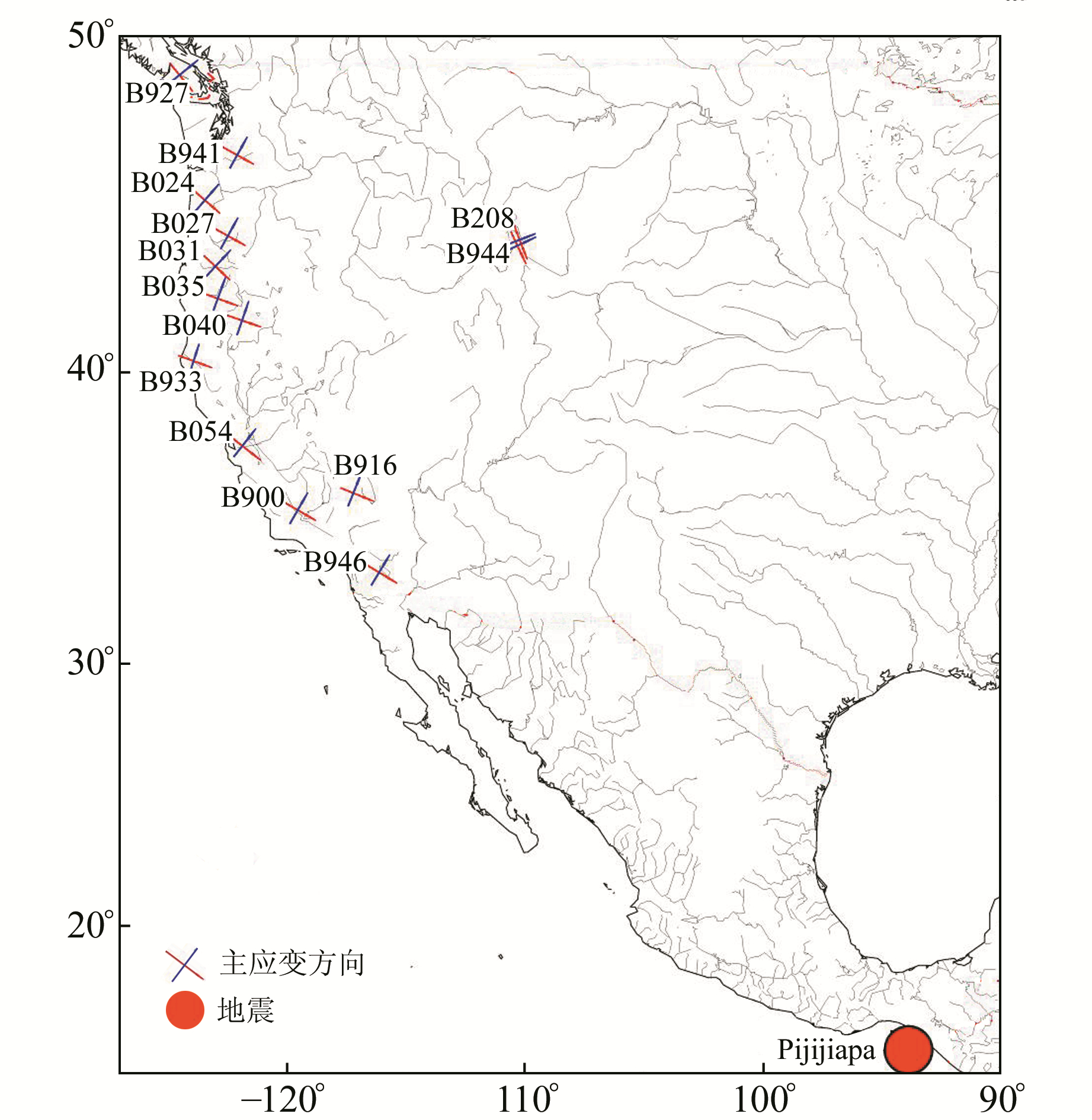图 6 钻孔的同震应变主方向 Fig. 6 Coseismic strain main direction
4 结语

1) 从震中距-走时拟合曲线可知，应变仪记录的地震波形与地震计记录的波形在信号响应上有较好的一致性。应变仪记录的地震波也能准确记录P、S等主要震相，其记录的波形射线参数符合理论射线参数值，钻孔应变仪记录的地震波是可靠的。

2) PBO钻孔应变仪体波波段的记录能力明显不如对面波的响应，其可能受1 sps采样限制，应变仪最早的设计是针对低频信号的。

3) 应变仪观测在面波段得到较完整的记录，较地震仪在面波段更有优势，可以结合应变仪和地震仪面波资料进行地壳结构研究，或者基于应变面波资料进行进一步研究。

4) 在远震条件下，PBO钻孔应变仪记录的地震信号其同震应变主方向指向震中，表明地震波传播到钻孔时应变仪记录的信号主体是地震波引起的形变。

  http://www.unavco.org/community/publications_and_reports/proposals/pbo/PBOwhitepaper.pdf (0)  Qiu Z H, Chi S L, Wang Z M, et al. The Strain Seismograms of P- and S-waves of a Local Event Recorded by Four-Gauge Borehole Strainmeter[J]. Earthquake Science, 2015, 28(3): 209-214 DOI:10.1007/s11589-015-0120-5 (0)  刘琦, 张晶, 晏锐, 等. 高采样率四分量钻孔应变同震响应分析[J]. 中国地震, 2013, 28(1): 57-67 (Liu Qi, Zhang Jing, Yan Rui, et al. The Analysis of Coseismic Response of 4-Component Borehole Strainmeters with High Sampling Rate[J]. Earthquake Research in China, 2013, 28(1): 57-67 DOI:10.3969/j.issn.1001-4683.2013.01.006) (0)  Barbour A J, Agnew D C. Detection of Seismic Signals Using Seismometers and Strainmeters[J]. Bulletin of the Seismological Society of America, 2012(6): 2484-2490 (0)  Barbour A J, Crowell B W. Dynamic Strains for Earthquake Source Characterization[J]. Seismological Research Letters, 2017(2): 354-370 (0)  Stockwell R G, Mansinha L, Lowe R P. Localization of the Complex Spectrum: The S Transform[J]. IEEE Transactions on Signal Processing, 1996(4): 998-1001 (0)  刘刚, 聂兆生, 方荣新, 等. 高频GNSS形变波的震相识别:模拟实验与实例分析[J]. 地球物理学报, 2014, 57(9): 2813-2825 (Liu Gang, Nie Zhaosheng, Fang Rongxin, et al. Recognition of Seismic Phases Record by High-Rate GNSS Measurements: Simulation and Case Studies[J]. Chinese J Geophys, 2014, 57(9): 2813-2825) (0)  孟庆筱, 吕健, 李进武, 等. 基于S变换的唐山四分量钻孔应力时频特征分析[J]. 大地测量与地球动力学, 2018, 38(11): 1202-1206 (Meng Qingxiao, Lü Jian, Li Jinwu, et al. Analysis of Time-Frequency Characteristics of Four-Components Borehole Stress at Tangshan Station Based on S-Transform[J]. Journal of Geodesy and Geodynamics, 2018, 38(11): 1202-1206) (0)  邱泽华, 石耀霖, 欧阳祖熙. 四分量钻孔应变观测的实地相对标定[J]. 大地测量与地球动力学, 2005(1): 118-122 (Qiu Zehua, Shi Yaolin, Ouyang Zuxi. Relative in-Situ Calibration of 4-Component Borehole Strain Observation[J]. Journal of Geodesy and Geodynamics, 2005(1): 118-122) (0)  Evelyn R. Tidal Calibration of Plate Boundary Observatory Borehole Strainmeters: Roles of Vertical and Shear Coupling[J]. Journal of Geophysical Research, 2010, B6 (0)  BarbourA J, Agnew D C, Wyatt F K. Coseismic Strains on Plate Boundary Observatory Borehole Strainmeters in Southern California[J]. Bulletin of the Seismological Society of America, 2015(1): 431-444 (0)  Kennett B L N, Engdahl E R. Travel Times for Global Earthquake Location and Phase Identification[J]. Geophysical Journal of the Royal Astronomical, 1991(2): 429-465 (0)
Analysis of Coseismic Response of PBO Borehole Strainmeters
CHEN Yifei1,2LÜ Pinji1,21. Key Laboratory of Earthquake Geodesy, Institute of Seismology, CEA, 40 Hongshance Road, Wuhan 430071, China;
2. Wuhan Base of Institute of Crustal Dynamics, CEA, 40 Hongshance Road, Wuhan 430071, China
Abstract: The PBO borehole strainmeter reaches 1 sps sampling. With the Mexico 8.1 earthquake on September 8, 2017 as an example, we analyze the seismic signals of 14 strainmeters and seismeters, showing that the seismic waves recorded by strainmeters meet the radiation law of transmission. The characteristics of the coseismic response of strainmeters and seismeters based on S-transform are analyzed for stations with different epicenter distances, and the coseismic responses of the strainmeters are very coherent with the seismeters' signals. The frequency of the strainmeters is mainly below 0.25 Hz. After seismic time series data calculation, we find that, in the coseismic phase, the main direction of strain points to the epicenter.
Key words: PBO; borehole strain; coseismic; strain main direction